# Introduction to Deep Learning and AI

## Introduction to Deep Learning and AI

In this article, I am going to give a brief Introduction to Deep Learning and AI. Please read our previous article where we discussed Multi-layer Perceptron Digit-Classifier using TensorFlow.

##### Introduction to Deep Learning and AI

Deep learning is a subfield of machine learning that deals with artificial neural networks, which are algorithms inspired by the structure and function of the brain. In other words, it mimics how human brains work. Deep learning algorithms are constructed similarly to the nervous system, with one neuron connecting to the next and transmitting information.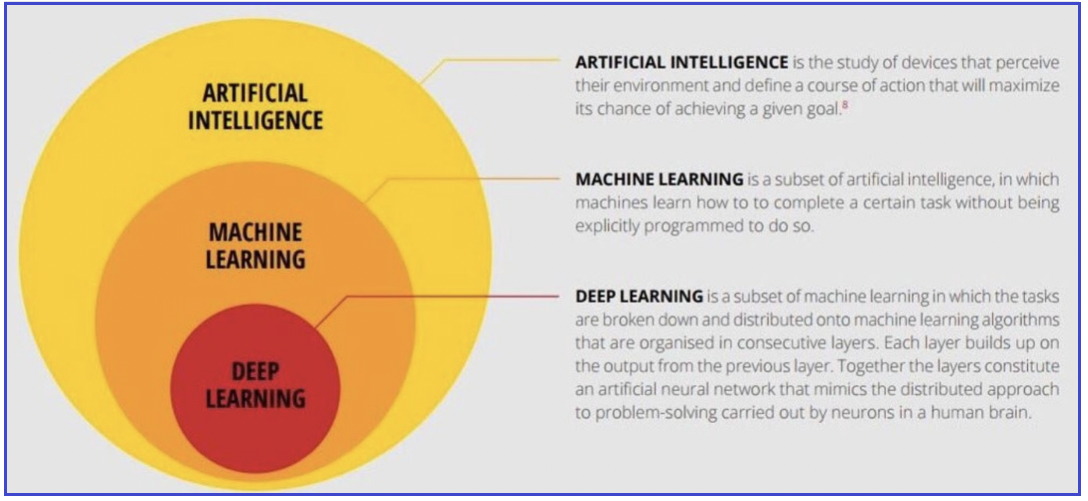Deep learning models operate in layers, with a typical model having at least three layers. Each layer takes information from the previous layer and passes it on to the next.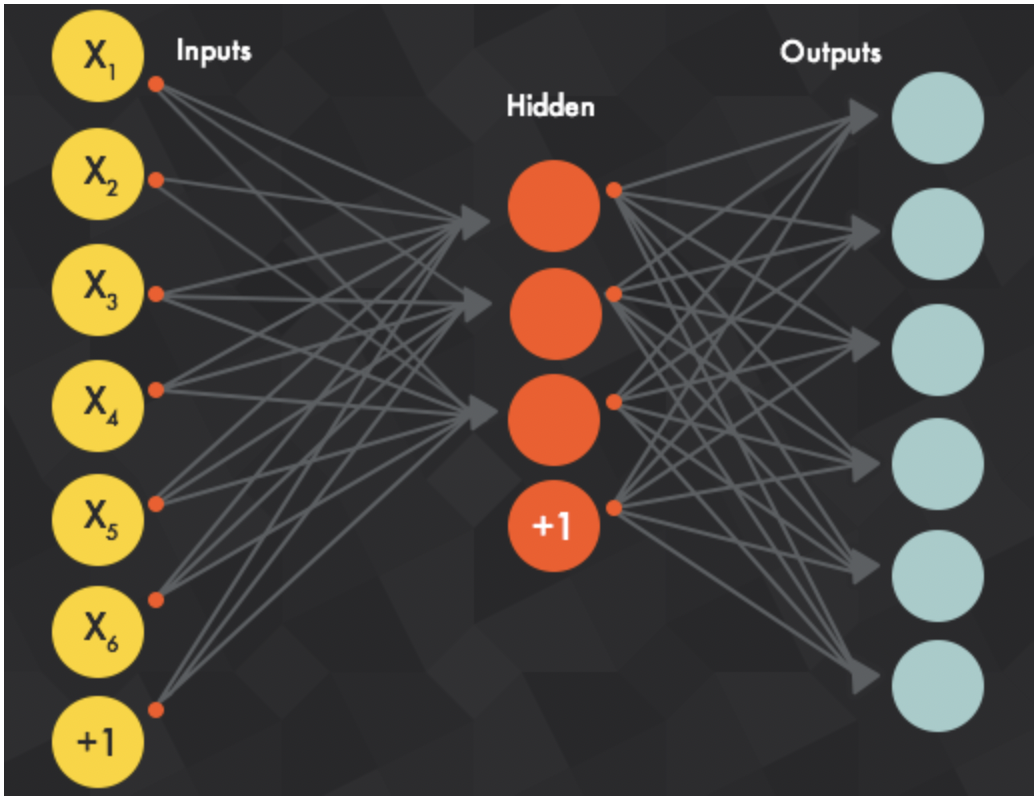Deep learning models tend to perform well with large amounts of data, but classic machine learning algorithms plateau at a certain point. One distinction between machine learning and deep learning models is in the domain of feature extraction. In machine learning, feature extraction is done by humans, but deep learning models figure it out on their own.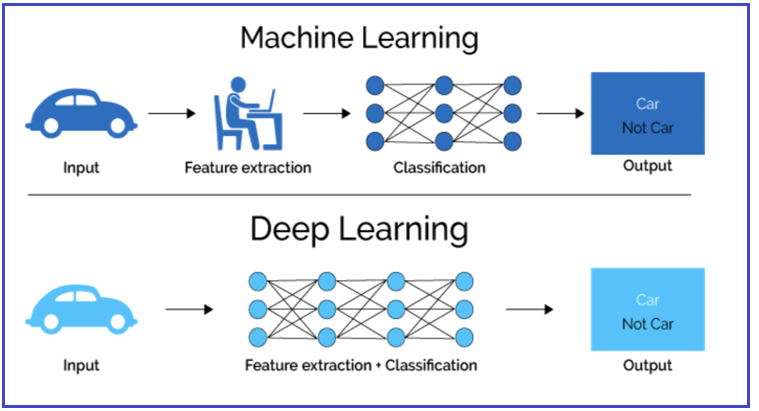Activation functions are functions that determine what the node’s output should be given the inputs. Because the activation function determines the actual output, we commonly refer to a layer’s outputs as its “activations.”

The Heaviside step function is one of the most basic activation functions. If the linear combination is smaller than zero, this method yields a 0. If the linear combination is positive or equal to zero, it returns a 1.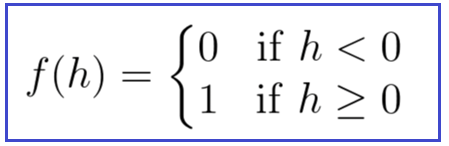###### Weights

When input data enters a neuron, it is multiplied by the weight value assigned to that input. The neuron in the above university example, for example, has two inputs, tests for test scores and grades, therefore it has two related weights that may be modified independently.

###### Using Weights

These weights begin as random numbers, and when the neural network learns more about what types of input data result in a student being admitted into a university, the network modifies the weights depending on any mistakes in classification caused by the prior weights. This is referred to as neural network training.

Remember that in the original linear equation, we may correlate weight with m(slope).

Y = Mx + B

###### Bias

Deep learning models’ learnable parameters are weights and biases. In the above linear equation, bias is given as b.

##### Neural Network

As previously stated, deep learning is a subfield of machine learning that deals with artificial neural networks, which are algorithms inspired by the structure and function of the brain. I’ll show you how to build a basic neural network using the example. Logistic regression is the approach used in the preceding example to segregate data using a single line. However, most of the time, we are unable to accurately classify the dataset using a single line.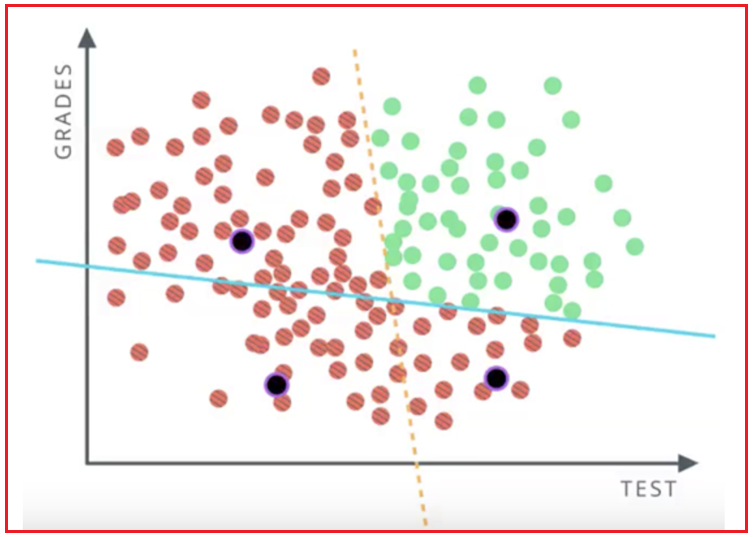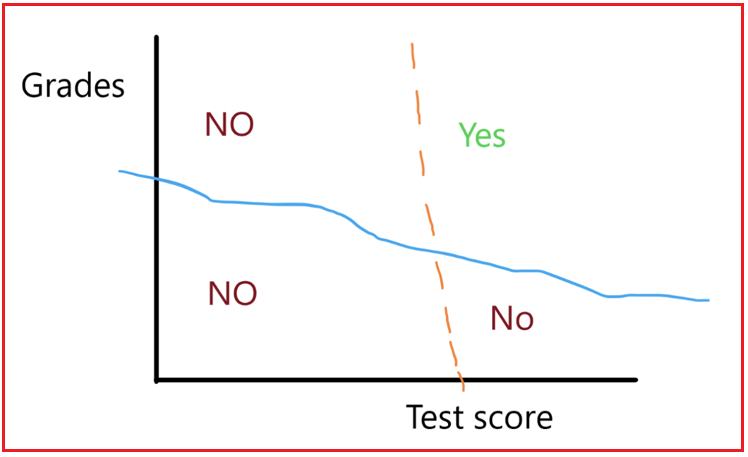In this scenario, everything below the blue line is “No (not passed)” and anything above it is “Yes (passed).” Similarly, anything on the left side is “No (not passed)” and anything on the right side is “Yes (passed)”.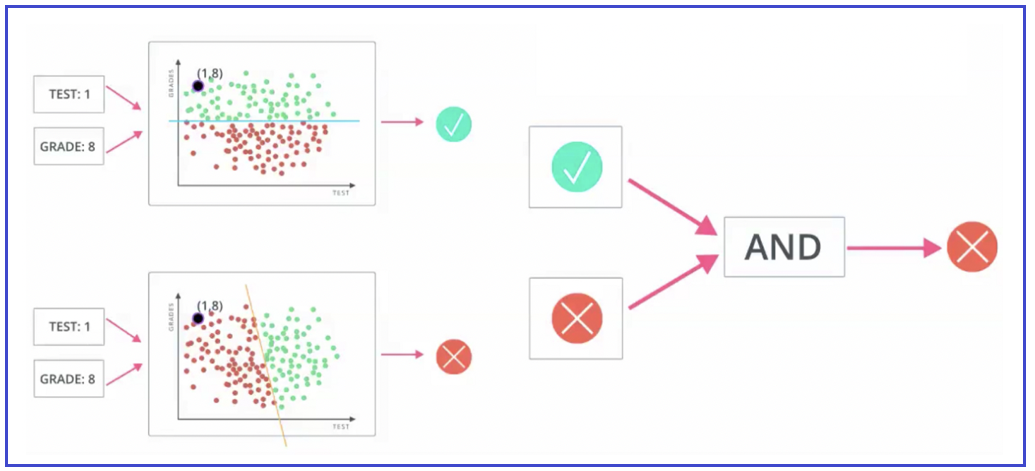Because neurons exist in the nervous system, we may describe each line as one neuron that is linked to neurons in the next layer as well as neurons in the same layer. In this scenario, the two lines are represented by two neurons. The image above depicts a rudimentary neural network in which two neurons collect input data and compute yes or no based on their condition before passing it to the second layer neuron, which concatenates the output from the previous layer. For this exact example, test score 1 and grade 8 input will result in “Not passed,” which is correct, however logistic regression output may result in “passed.”

To summarize, we may improve the model’s accuracy by combining many neurons in different layers. This is the foundation of a neural network.

A basic network is depicted in the diagram below. The input h is formed by the linear combination of the weights, inputs, and bias, which then goes through the activation function f(h), yielding the final output, denoted y.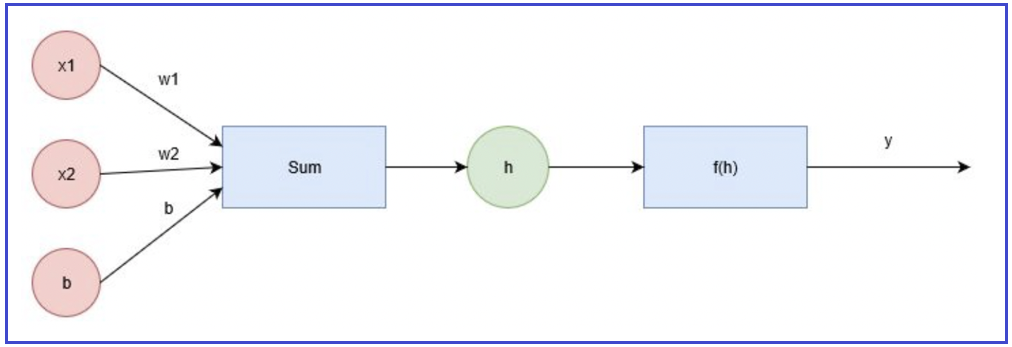The beautiful thing about this design, and what allows neural networks to operate, is that the activation function, f(h), can be any function other than the step function presented before. If you allow f(h)=h, for example, the output will be the same as the input. The network’s output is now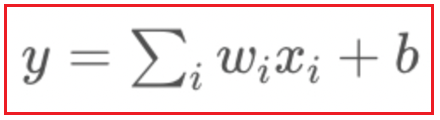You should be familiar with this equation because it is the same as the linear regression model! The logistic (also known as the sigmoid), tanh, and softmax functions are examples of activation functions.

The sigmoid function has a range of 0 to 1, and its output can be read as a likelihood of success. Using a sigmoid as the activation function produces the same formulation as logistic regression. Finally, we can state the output of the basic neural network based on sigmoid as follows: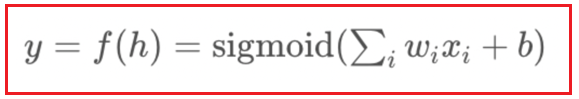##### The Brain vs Neuron

In recent years, “deep learning” AI models have been marketed as “functioning like the brain,” since they are made up of artificial neurons that imitate those found in real brains.

It is pointless to reiterate that AI is on the rise. And one key contributor to this surge has been the development of artificial neural networks (ANN), which have been widely employed to create models for a wide range of scenarios. Since its origin, an ANN has undergone several modifications, each with its unique utility. However, because it is so simple to apply (especially with Keras in our arsenal), we frequently overlook its origins. The concept of an artificial neural network (ANN) is based on how the brain functions, or so its creators claim. ANN was created with the human brain’s functioning in mind.

There has been no dearth of research in the field of studying the brain either. We know more about our brain now more than we knew a century ago. We have come a long way since then and maybe now is the right time to check how accurate that analogy between an ANN and an actual brain is.

The correct way of doing it is to first study human behavior. The human brain has a biological neural network that has billions of interconnections. As the brain learns, these connections are either formed, changed, or removed, similar to how an artificial neural network adjusts its weights to account for a new training example. This is the reason why it is said that practice makes one perfect since a greater number of learning instances allows the biological neural network to become better at whatever it is doing.

This is why it is claimed that practice makes perfect because a larger number of learning instances permits the biological neural network to improve at whatever it is doing. Only a subset of neurons in the nervous system is activated depending on the stimulation. A neuron is seen in the diagram. A dendritic network receives information from neighboring neurons and sends it to the nucleus. The incoming impulses are aggregated in the nucleus, and if they surpass a threshold, the axon sends a signal down to the other neurons.

Now let us compare it to an artificial neural network. The existence of neurons as the most basic unit of the nervous system is the most evident resemblance between a neural network and the brain. However, the way neurons process input differs in both circumstances. We know from our study of the biological brain network that input comes from dendrites and output comes from axons. These have quite distinct methods of processing input. According to research, dendrites apply a non-linear function to the input before passing it to the nucleus.

In an artificial neural network, on the other hand, input is directly supplied to a neuron, and output is likewise directly obtained from the neuron.

While a neuron in an artificial neural network may provide a continuous series of outputs, a neuron in an artificial neural network can only produce a binary output of a few tens of millivolts.

The signal is passed and aggregated in the following manner. The membrane of a neuron has a resting potential of about -70mV. If the nucleus’s aggregated signals reach a specific threshold, the axon sends a high voltage known as the action potential (hence the binary). For example, if the threshold is -55mV and each neuron emits 5mV, this neuron must be activated by at least three neurons in order to carry information on. The graph below demonstrates this. After the threshold is reached and the signal is sent by the axon, the cell may be unresponsive for a short amount of time known as the absolute refractory period.

Because information transmission in a biological neural network is binary, research has demonstrated that the nervous system stores information in the frequency at which impulses are transferred, where the average frequency at which impulses are transmitted becomes crucial. According to some studies, information is sent utilizing temporal encoding, in which the time at which the impulses are received is more relevant. In any scenario, the method is markedly different from some of the strategies employed in artificial neural networks to convey information between neuron layers, such as one-hot encoding of categorical information.

Learning at a very abstract level is comparable in both systems. However, the technique of instruction differs. Gradient descent is used by artificial neural networks to reduce a loss function and attain the global minimum. Biological neural networks use a unique learning approach. Gradient descent needed backpropagation in artificial neural networks, which is only attainable to the extent of one neuron in a biological neural network. Biological neural networks employ the Hebbian learning process, in which the effectiveness of one neuron’s ability to stimulate another neuron is improved by as many learning examples as feasible. Spike-Dependent-Timing If input spikes in the first neuron occur just before output spikes in the second neuron, plasticity strengthens the link between neurons.

Generalization, or the ability to abstract knowledge from what one has previously learned, is an extremely useful capability that allows rapid problem-solving across domains through minor weight adjustment — a process known as fine-tuning — which is a neural network’s solution to transfer learning and domain adaptation problems. One reason why if a person has one talent, it doesn’t take long to learn another is that not all neural connections require rewiring.

One significant distinction between an artificial neural network and the brain is that the neural network will provide the same result for the same input whereas the brain may falter. It may not always respond in the same manner to the same input, which is referred to as a human mistake in business.

Many new variants in artificial neural networks have been presented recently. A convolutional neural network is one that processes pictures, with each layer using a convolution procedure followed by various operations on images that compress or expand the dimensions of the image, allowing the network to capture just the information that matter.

It was observed that neurons in the primary visual cortex respond to certain environmental elements such as edges. There are two types of cells: simple cells and complicated cells. Simple cells only reacted in one orientation, whereas complex cells responded in several orientations. It was determined that complex cells aggregated inputs from simple cells, resulting in spatial invariance in complex cells. A convolutional neural network was inspired by this.

To understand how sensory information is encoded in the brain, encoding models are used to predict brain activity in response to sensory stimuli. Typically, encoding models use a nonlinear transformation of inputs to features and a linear convolution of features to responses.

There are several more distinctions. For example, the human brain has around 86 billion neurons. Normal artificial neural networks have fewer than 1000 neurons, which is nowhere near enough. According to research, organic brain networks consume roughly 20W of electricity, but artificial neural networks consume over 300W.

The world is moving closer to an era of increased artificial intelligence as the gap between the human brain and neural networks decreases.

In the next article, I am going to discuss Deep Learning with Tensorflow. Here, in this article, I try to give a brief introduction to Deep Learning and AI. I hope you enjoy this introduction to Deep Learning and AI article. Please post your feedback, suggestions, and questions about this introduction to Deep Learning and AI article.